$$\require{physics}$$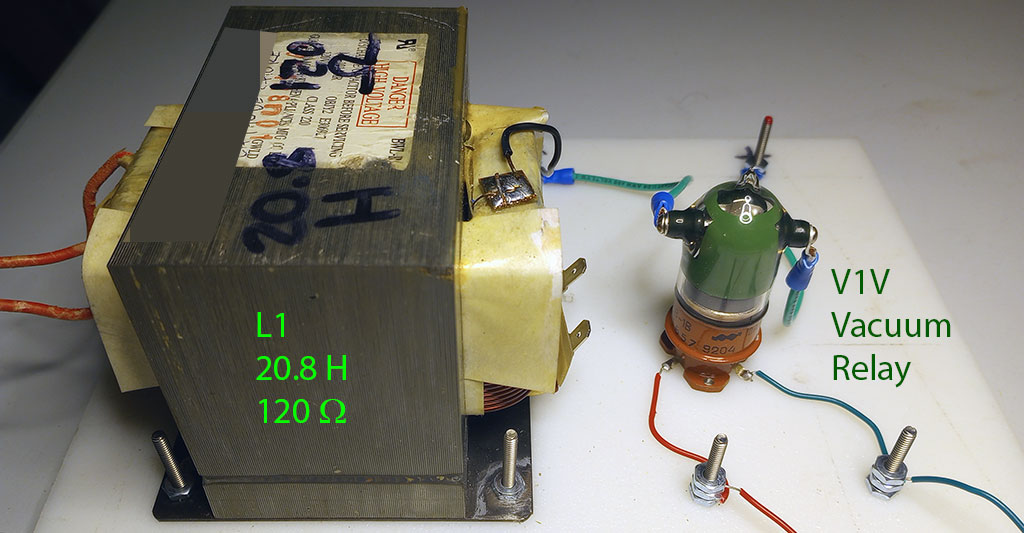# Pulse Response: Coulomb Potential Pulses

When the switch is closed, current begins flowing through the inductor. When the switch opens, the "momentum" of the flux in the coil pulls the voltage on the pipe very low, very suddenly. SPICE says -110 kV. It's like a water hammer.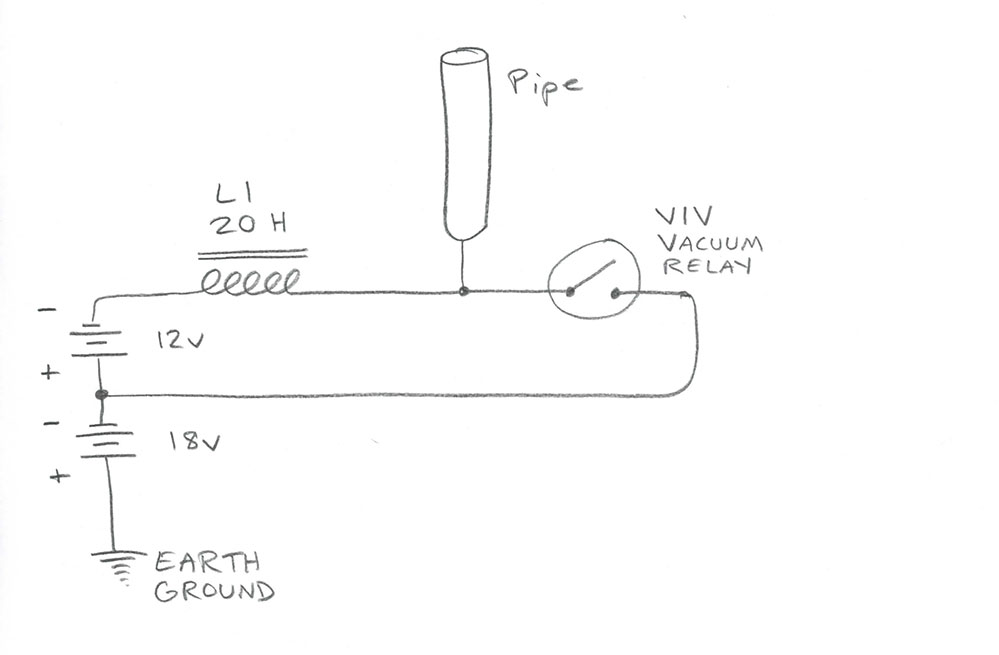Due to the large amount of inductance (20 Henrys), it takes 1 to 2 seconds for the current to ramp up to its maximum level. The timing circuit I made to control the relay closes it for about 1 second, then opens it for about 2. So the pulses we're generating are pretty far apart in time.

The whole circuit is charged negatively with respect to Earth ground. That's what the 18v battery is for. The Coulomb Potential of the pipe is varied with respect to time, but it always remains negative. The magnitude of the Coulomb Potential varies, but its sign does not change.

I am using a V1V (B1B) vacuum relay as my switch. It works well and does not arc over when it opens (breaks the circuit). However the coil I'm using, the secondary of a microwave oven transformer, sometimes arcs when the relay opens. The arc looks like purple lightning and is about 1 cm long. I figure that's about 30kV, since the rule of thumb is 3kV per mm of arc. To avoid arcing, I turned down the voltage to 12v, instead of 100v. It might also help to immerse the coil in oil.

You can wire the pipe in series, between the switch and inductor, if you like.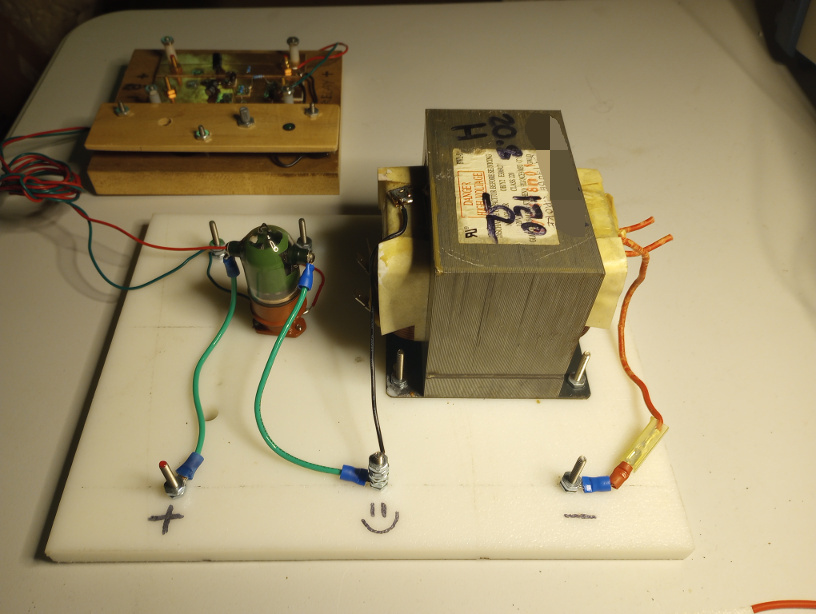$$I = \frac{V}{R} \left( 1 - e^{-tR/L} \right)$$

Click here for the Simulation of the current through this LR circuit.

## Coulomb Potential of a Charged Cylindrical Shell vs. Its Electric Field

The Coulomb Potential in large inside the cylinder, but the Electric Field is nearly zero in there.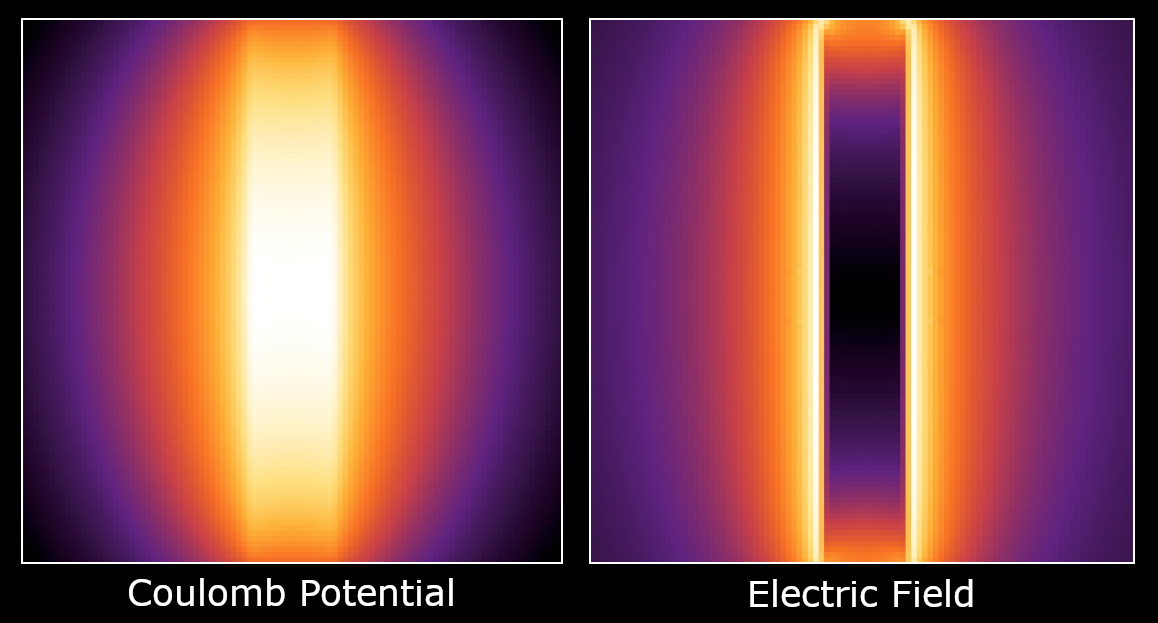If you were climbing Mt. Hood, your altitude at any given point is your Coulomb Potential. The steepness of the mountain at any point is your Electric Field. The Electric Field is the derivative of the Potential.

Let's say you climb to the top of a high, flat mesa. Your Coulomb Potential is large, because you're high up. But your E Field is zero, because the top of the mesa is flat. It is possible to have large Potential but small or zero E Field.

The Coulomb Potential of a Uniformly Charged Cylindrical Shell, in Cylindrical Coordinates

$$U(r,z,R,L) = \frac{k_e Q}{L} \int_0^\infty{ J_0(kr) J_0(kR) g(k,z,L) dk}$$

$$g(k,z,L) = \int_0^L{ e^{-k \lvert z-z' \rvert } dz'}$$

$$g(k,z,L) = \frac{1}{k} \left( 2 - e^{-kz} - e^{k(z-L)} \right)$$

$$U(r,z,R,L) = \frac{k_e Q}{L} \int_0^\infty{ J_0(kr) J_0(kR) \frac{1}{k} \left( 2 - e^{-kz} - e^{k(z-L)} \right) dk}$$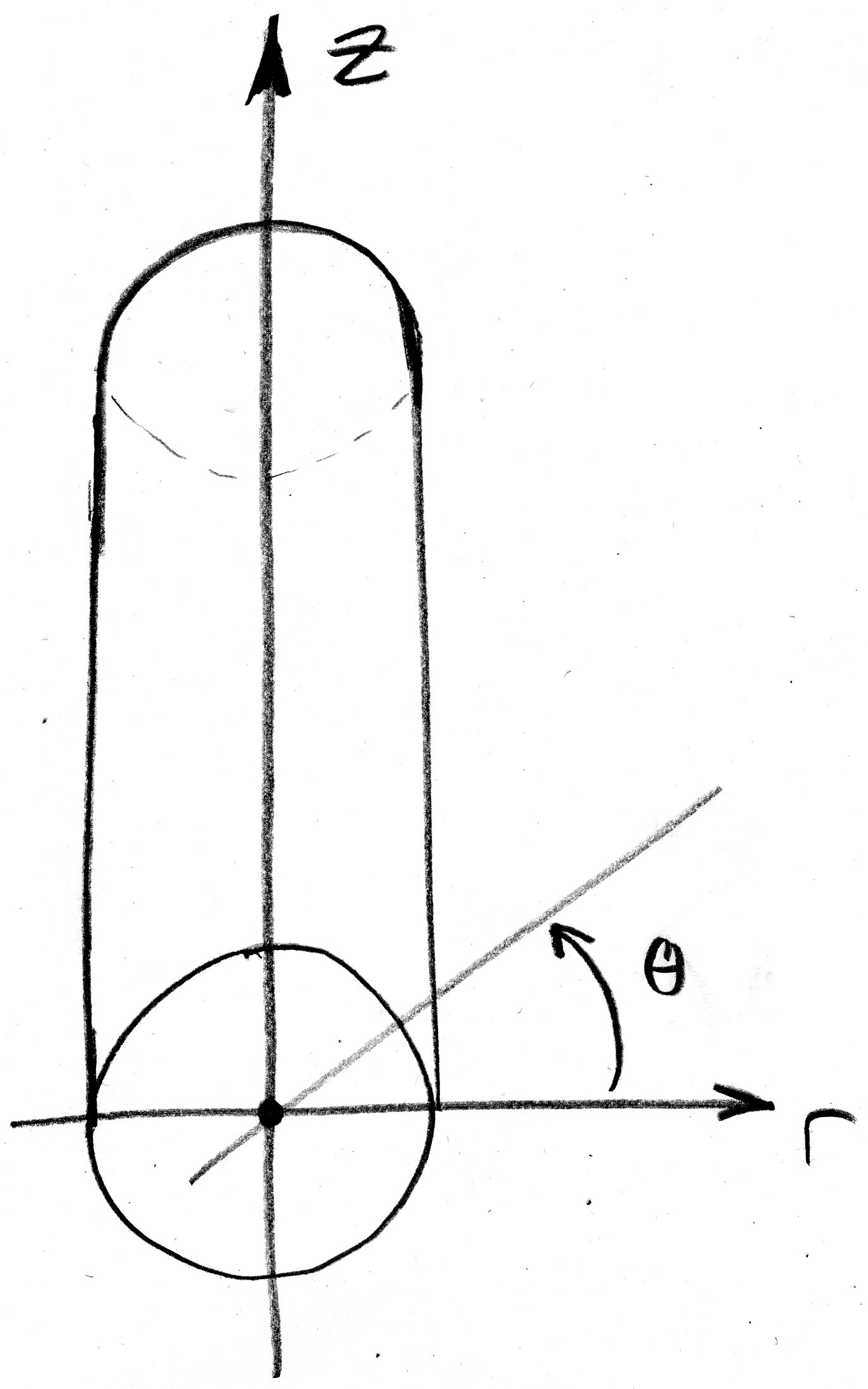Where:
L = Length of Cylinder
r = r-coordinate of the point where potential is being measured
z = z-coordinate of the point
(Note: The theta-coordinate is irrelevant)
Q = Charge distributed over cylinder (Coulombs)
k_e = 8.988 * 10^9 N m^2 / C^2 Coulomb's Constant
J0() = Bessel Function of the First Kind, of order zero.
e = Base of Natural Logarithms
k = dummy variable of integration

Let's look at the Potential down the length of the cylinder, from one end to the other. Here r = 0, we are in the center, and we vary z from zero to the length of the cylinder.

We can clearly see the Potential is large near the center of the cylinder. Now let's slice our cylinder another way: Perpendicular to the z-axis.

Each color represents a different z-coordinate. Blue (lowest) is near the end of the cylinder. Brown (highest) is at the center. For each z-coordinate slice, the Potential is more or less level inside the cylinder (the plateaux), and drops off once we pass outside the cylinder wall.

Imagine a plane slicing the cylinder in half long-ways. This plane lies on the z-axis. We can spin the plane around the z-axis by any angle theta, and it won't make any difference. We compute the Potential on this r-z plane, and it will look the same for any angle theta.

## Electric Field

The Electric Field is the (negative) Gradient of the Potential. In Cylindrical coordinates:

$$\vb{E} = - \grad{U}$$

$$\grad{ U } = \pdv{U}{r} \vectorunit{r} + \frac{1}{r} \pdv{U}{\phi} \vectorunit{\phi} + \pdv{U}{z} \vectorunit{z}$$

For our purposes, we can disregard the angular term, because we are working only in the r-z plane.

$$\vb{E} = - \pdv{U}{r} \vectorunit{r} - \pdv{U}{z} \vectorunit{z}$$

The Electric Field is a Vector Field, but we can think of it as a Scalar Field, if we just consider the Magnitude of the vectors. We know which way they point: Away from the cylinder. Let's take a look at what the Electric Field looks like.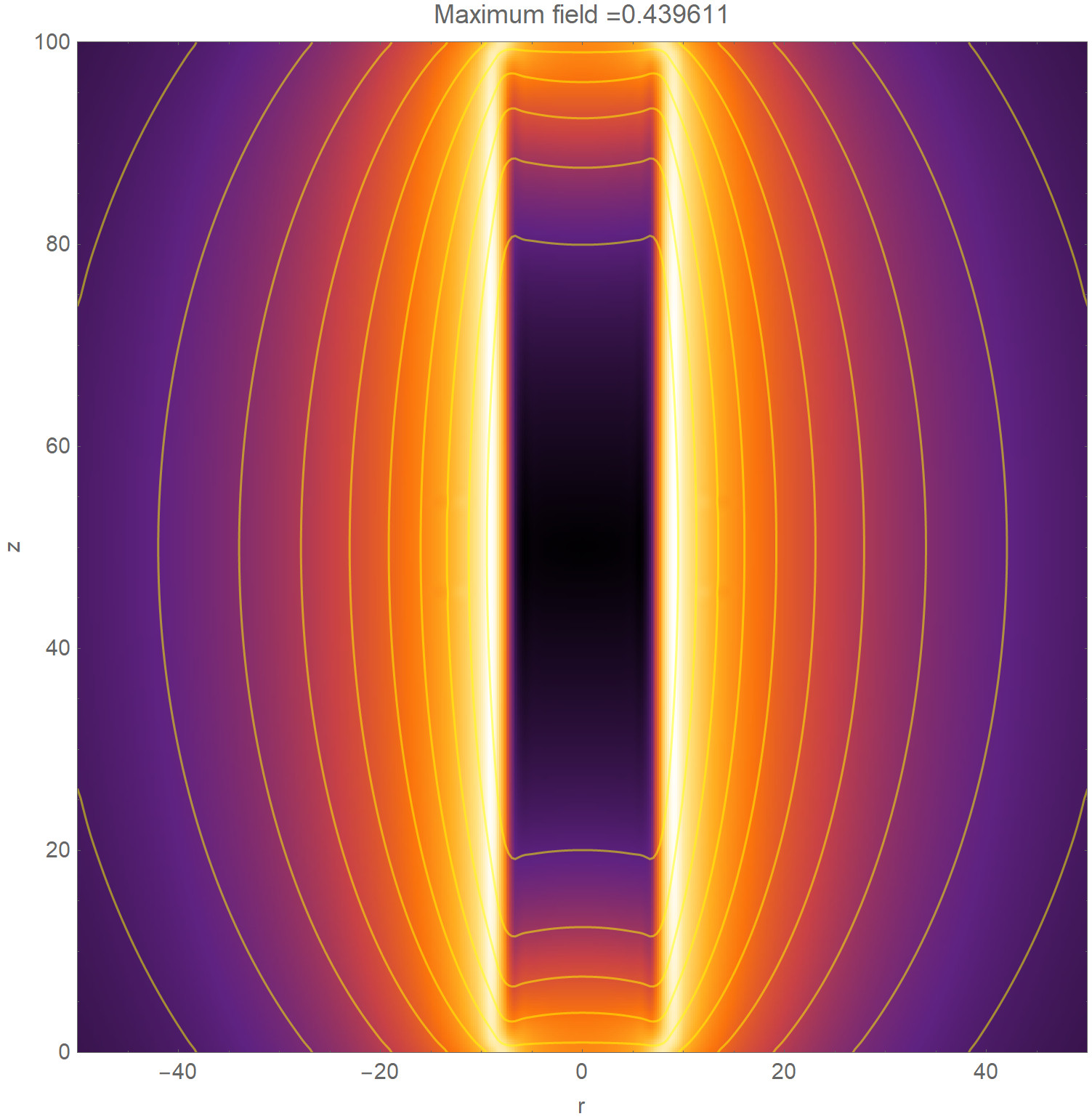We can also see the field lines if you prefer.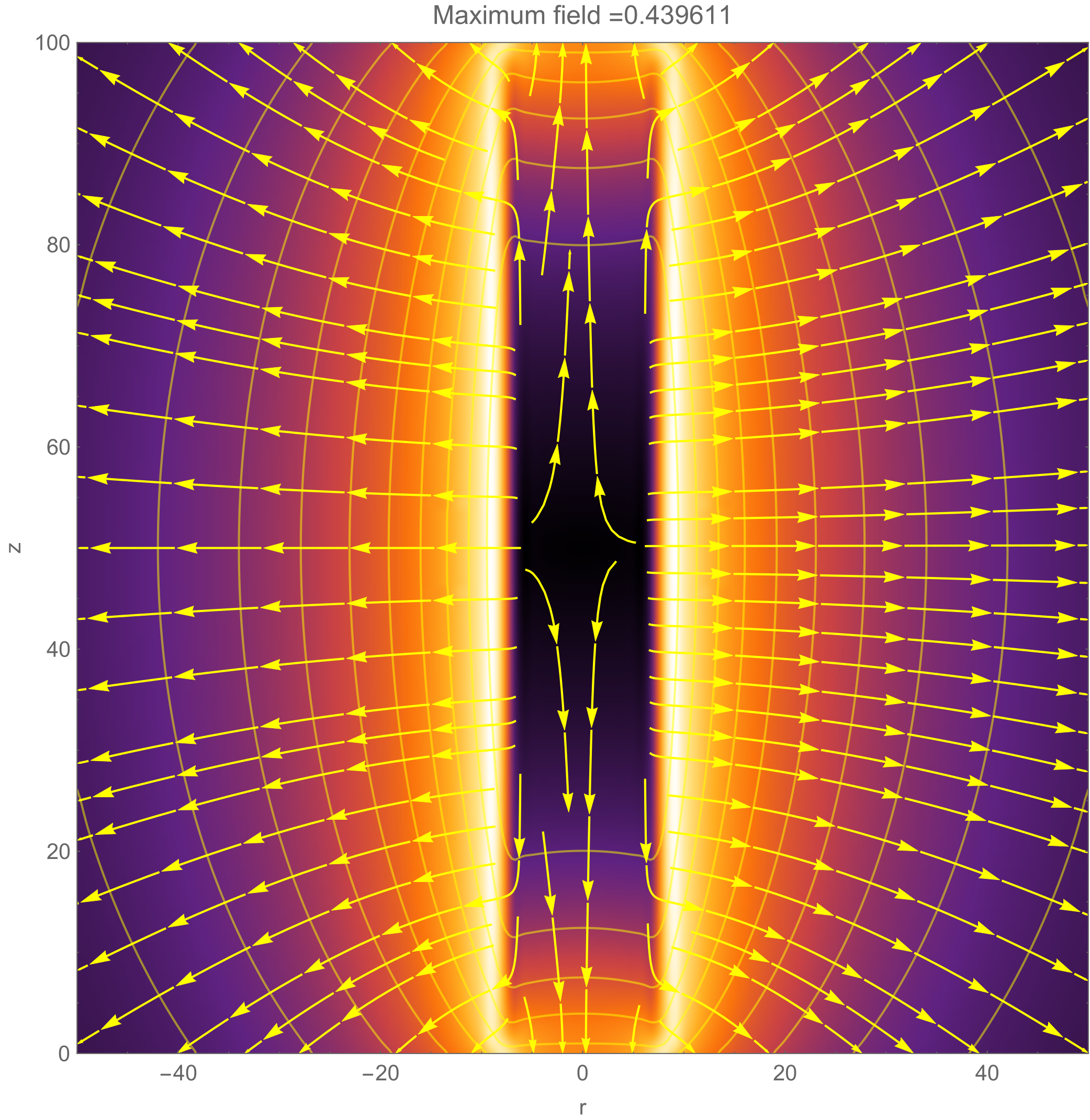You can download the Mathematica notebooks used to create these images. I have also included PDF copies of the notebooks, and CSV copies of the data files, so you can see what's going on, and work with the data, even if you don't have Mathematica.

## The Coulomb Potential is More Fundamental than the Electric Field, and it has Physical Existence.

It occurred to me, as I was looking at the images of the Coulomb Potential and the Electric Field, that the Coulomb Potential is more fundamental than the E field. If you think of the image of the Potential as a 2-dimensional array of values, you can take the Gradient (the Derivative) of the Potential to get the E Field. But if you integrate the E Field, you do not recover the original Potential.

In Maxwell's Equations, we deal only with the Electric and Magnetic Fields, not the Potentials. The Coulomb Potential, and the Magnetic Vector Potential, are thought of merely as useful mathematical concepts, which have no existence in physical reality. But I thought, "The Coulomb Potential must be something real, in real life."

Yakir Aharonov and David Bohm thought so as well. They showed that the Coulomb Potential, and the Magnetic Vector Potential, both have real physical existence, and can affect particles such as electrons. To quote from their paper, "Significance of Electromagnetic Potentials in the Quantum Theory", 1959, p. 490, they conclude:

In classical mechanics, we recall that potentials cannot have such significance because the equation of motion involves only the field quantities themselves. For this reason, the potentials have been regarded as purely mathematical auxiliaries, while only the field quantities were thought to have a direct physical meaning.
In quantum mechanics, the essential difference is that the equations of motion of a particle are replaced by the Schrodinger equation for a wave. This Schrodinger equation is obtained from a canonical formalism, which cannot be expressed in terms of the fields alone, but which also requires the potentials. Indeed, the potentials play a role, in Schrodinger's equation, which is analogous to that of the index of refration in optics, The Lorentz force does not appear anywhere in the fundamental theory, but appears only as an approximation holding in the classical limit. It would therefore seem natural at this point to propose that, in quantum mechanics, the fundamental physical entities are the potentials, while the fields are derived from them by differentiations.
The main objection that could be raised against the above suggestion is grounded in the gauge invariance of the theory. In other words, if the potentials are subject to the transformation [...], then all the known physical quantities are left unchanged. As a result, the same physical behavior is obtained from any two potentials, A„(x) and A„'(x), related by the above transformation. This means that insofar as the potentials are richer in properties than the fields, there is no way to reveal this additional richness. It was therefore concluded that the potentials cannot have any meaning, except insofar as they are used mathematically, to calculate the fields.
We have seen from the examples described in this paper that the above point of view cannot be maintained for the general case. Of course, our discussion does not bring into question the gauge invariance of the theory. But it does show that in a theory involving only local interactions (e.g., Schrodinger's or Dirac's equation, and current quantum-mechanical field theories), the potentials must, in certain cases, be considered as physically effective, even when there are no fields acting on the charged particles.

## References

1. Ciftja, Orion. Batle, Joseph. "Coulomb Potential and Energy of a Uniformly Charged Cylindrical Shell." 5 September, 2018
2. Aharonov, Yakir. Bohm, David. "Significance of Electromagnetic Potentials in the Quantum Theory". The Physical Review, Volume 115, Number 3, August 1st, 1959.